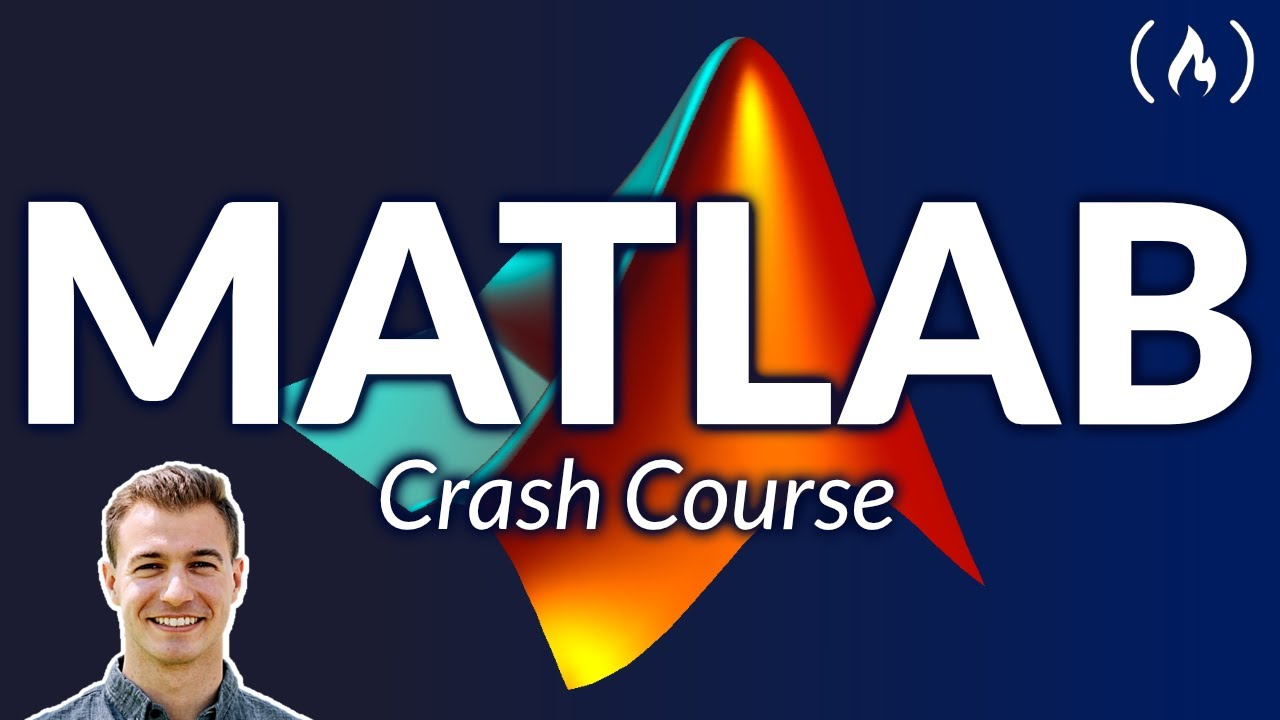# MATLAB Crash Course for BeginnersLearn the fundametnals of MATLAB in this tutorial for engineers, scientists, and students. MATLAB is a programming language and software suite used for data analysis, scientific computing, and visualization that is widely used in academia and industry.

Learn the fundametnals of MATLAB in this tutorial for engineers, scientists, and students. MATLAB is a programming language and software suite used for data analysis, scientific computing, and visualization that is widely used in academia and industry.

⭐️ Contents ⭐️
⌨️(0:00:00) Intro
⌨️(0:00:19) MATLAB IDE
⌨️(0:01:33) Variables & Arithmetic
⌨️(0:14:19) Matrices, Arrays, & Linear Algebra
⌨️(0:24:54) The Index
⌨️(0:31:16) Example 1 - Equations
⌨️(0:45:09) Anonymous Functions
⌨️(0:47:57) Example 2 - Plotting
⌨️(1:05:29) Example 3 - Logic
⌨️(1:20:24) Example 4 - Random & Loops
⌨️(1:26:07) Sections
⌨️(1:28:20) For Loops
⌨️(1:40:09) Calculation Time
⌨️(1:42:57) Naming Conventions
⌨️(1:45:09) File Naming
⌨️(1:45:54) While Loop
⌨️(1:50:16) Custom Function
⌨️(1:56:47) Have a good one ;)